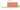# د.ع.‏

## Iraqi Dinar

Iraq's official currency is the dinar. It is issued by the Central Bank of Iraq and divided into 1,000 fils, yet since inflation became a problem in the 1990s, the fils have lost their usefulness. The US Dollar was at US\$1 = 1462.5 dinars on June 19, 2022.

#### how has the value of the currency changed in the last year?## USD 1 = IQD 1,310.3

How much is10 US dollars worth inIraqi dinars?
At the current exchange rate, 10 US dollars is worth 13,102.5 Iraqi dinars
How much is50 US dollars worth inIraqi dinars?
At the current exchange rate, 50 US dollars is worth 65,512.5 Iraqi dinars
How much is100 US dollars worth inIraqi dinars?
At the current exchange rate, 100 US dollars is worth 131,025 Iraqi dinars
How much is500 US dollars worth inIraqi dinars?
At the current exchange rate, 500 US dollars is worth 655,125 Iraqi dinars
How much is2,000 US dollars worth inIraqi dinars?
At the current exchange rate, 2,000 US dollars is worth 2,620,500 Iraqi dinars
How much is10 US dollars worth inIraqi dinars?
At the current exchange rate, 10 US dollars is worth 13,102.5 Iraqi dinars
How much is50 US dollars worth inIraqi dinars?
At the current exchange rate, 50 US dollars is worth 65,512.5 Iraqi dinars
How much is100 US dollars worth inIraqi dinars?
At the current exchange rate, 100 US dollars is worth 131,025 Iraqi dinars
How much is500 US dollars worth inIraqi dinars?
At the current exchange rate, 500 US dollars is worth 655,125 Iraqi dinars
How much is2,000 US dollars worth inIraqi dinars?
At the current exchange rate, 2,000 US dollars is worth 2,620,500 Iraqi dinars